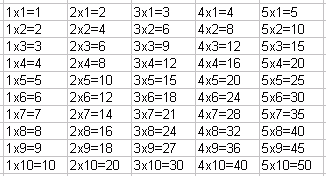Name: ___________________Date:___________________

 Email us to get an instant 20% discount on highly effective K-12 Math & English kwizNET Programs!

### Grade 2 - Mathematics7.4 Multiplication 0-5

 Practice counting by twos, threes, fours and fives.Method: Step 1: Each product is the result of repeated addition. Step 2: Find the product by multiplying the numbers. Example: 2*5 Answer: 10 Directions: Find the product of two numbers for the following. Also write at least ten examples of your own and multiplication tables of 0 - 5.Name: ___________________Date:___________________

### Grade 2 - Mathematics7.4 Multiplication 0-5

 Q 1: 4 x 3 =712813 Q 2: 3 x 9 =25272428 Q 3: 2 x 12 =22242520 Q 4: 5 x 5 =20255535 Q 5: 2 x 11 =2291320 Q 6: 5 x 5 =2010525 Q 7: 3 x 7 =20104none of the above Q 8: 0 x 5 =10015 Question 9: This question is available to subscribers only! Question 10: This question is available to subscribers only!

#### Subscription to kwizNET Learning System costs less than \$1 per month & offers the following benefits:

• Unrestricted access to grade appropriate lessons, quizzes, & printable worksheets
• Instant scoring of online quizzes
• Progress tracking and award certificates to keep your student motivated
• Unlimited practice with auto-generated 'WIZ MATH' quizzes